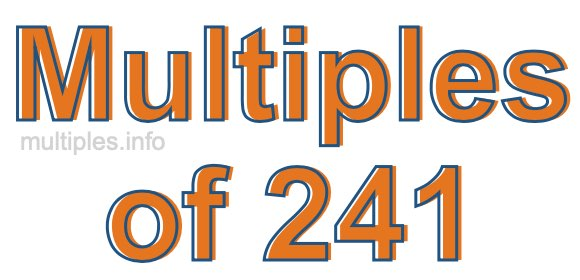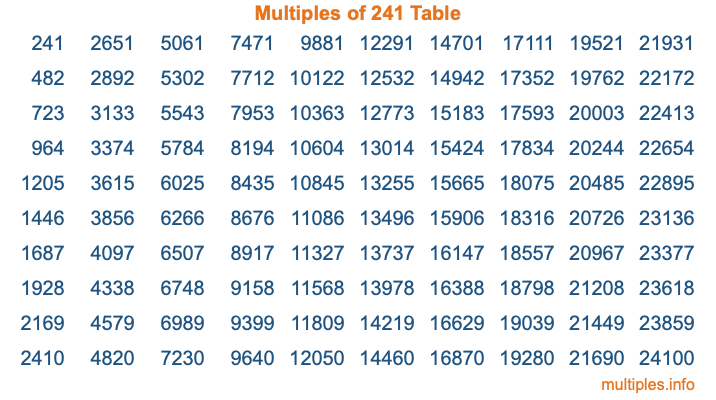Multiples of 241Welcome to the Multiples of 241 page. Here we will first teach you everything you will ever need to know about the multiples of 241, and then give you a study guide summary of everything we taught you to make sure you remember it all. Use this page to look up facts and learn information about the multiples of 241. This page will make you a multiples of two hundred forty-one expert!

Definition of Multiples of 241
Multiples of 241 are all the numbers that when divided by 241 equal an integer. Each of the multiples of 241 are called a multiple. A multiple of 241 is created by multiplying 241 by an integer.

Therefore, to create a list of multiples of 241, you start with 1 multiplied by 241, then 2 multiplied by 241, then 3 multiplied by 241, and so on for as long as you want. Thus, the list of the first five multiples of 241 is 241, 482, 723, 964, and 1205. To see a larger list of multiples of 241, see the printable image of Multiples of 241 further down on this page. We also have a category where you can choose any nth multiple of 241.

Multiples of 241 Checker
The Multiples of 241 Checker below checks to see if any number of your choice is a multiple of 241. In other words, it checks to see if there is any number (integer) that when multiplied by 241 will equal your number. To do that, we divide your number by 241. If the the quotient is an integer, then your number is a multiple of 241.

Is  a multiple of 241?

Least Common Multiple of 241 and ...
A Least Common Multiple (LCM) is the lowest multiple that two or more numbers have in common. This is also called the smallest common multiple or lowest common multiple and is useful to know when you are adding our subtracting fractions. Enter one or more numbers below (241 is already entered) to find the LCM.

Check out our LCM Calculator if you need more details about the Least Common Multiple or if you need the LCM for different numbers for adding and subtraction fractions.

nth Multiple of 241
As we stated above, 241 is the first multiple of 241, 482 is the second multiple of 241, 723 is the third multiple of 241, and so on. Enter a number below to find the nth multiple of 241.

th multiple of 241

Multiples of 241 vs Factors of 241
241 is a multiple of 241 and a factor of 241, but that is where the similarities end. All postive multiples of 241 are 241 or greater than 241. All positive factors of 241 are 241 or less than 241.

Below is the beginning list of multiples of 241 and the factors of 241 so you can compare:

Multiples of 241: 241, 482, 723, 964, 1205, etc.

Factors of 241: 1, 241

As you can see, the multiples of 241 are all the numbers that you can divide by 241 to get a whole number. The factors of 241, on the other hand, are all the whole numbers that you can multiply by another whole number to get 241.

It's also interesting to note that if a number (x) is a factor of 241, then 241 will also be a multiple of that number (x).

Multiples of 241 vs Divisors of 241
The divisors of 241 are all the integers that 241 can be divided by evenly. Below is a list of the divisors of 241.

Divisors of 241: 1, 241

The interesting thing to note here is that if you take any multiple of 241 and divide it by a divisor of 241, you will see that the quotient is an integer.

Multiples of 241 Table
Below is an image of the first 100 multiples of 241 in a table. The table is in chronological order, column by column. The first column has the first ten multiples of 241, the second column has the next ten multiples of 241, and so on.The Multiples of 241 Table is also referred to as the 241 Times Table or Times Table of 241. You are welcome to print out our table for your studies.

Negative Multiples of 241
Although not often discussed or needed in math, it is worth mentioning that you can make a list of negative multiples of 241 by multiplying 241 by -1, then by -2, then by -3, and so on, to get the following list of negative multiples of 241:

-241, -482, -723, -964, -1205, etc.

Multiples of 241 Summary
Below is a summary of important Multiples of 241 facts that we have discussed on this page. To retain the knowledge on this page, we recommend that you read through the summary and explain to yourself or a study partner why they hold true.

There are an infinite number of multiples of 241.

A multiple of 241 divided by 241 will equal a whole number.

241 divided by a factor of 241 equals a divisor of 241.

The nth multiple of 241 is n times 241.

The largest factor of 241 is equal to the first positive multiple of 241.

241 is a multiple of every factor of 241.

241 is a multiple of 241.

A multiple of 241 divided by a divisor of 241 equals an integer.

241 divided by a divisor of 241 equals a factor of 241.

Any integer times 241 will equal a multiple of 241.

Multiples of a Number
Here you can get the multiples of another number, all with the same attention to detail as we did for multiples of 241 on this page.

Multiples of
Multiples of 242
Did you find our page about multiples of two hundred forty-one educational? Do you want more knowledge? Check out the multiples of the next number on our list!